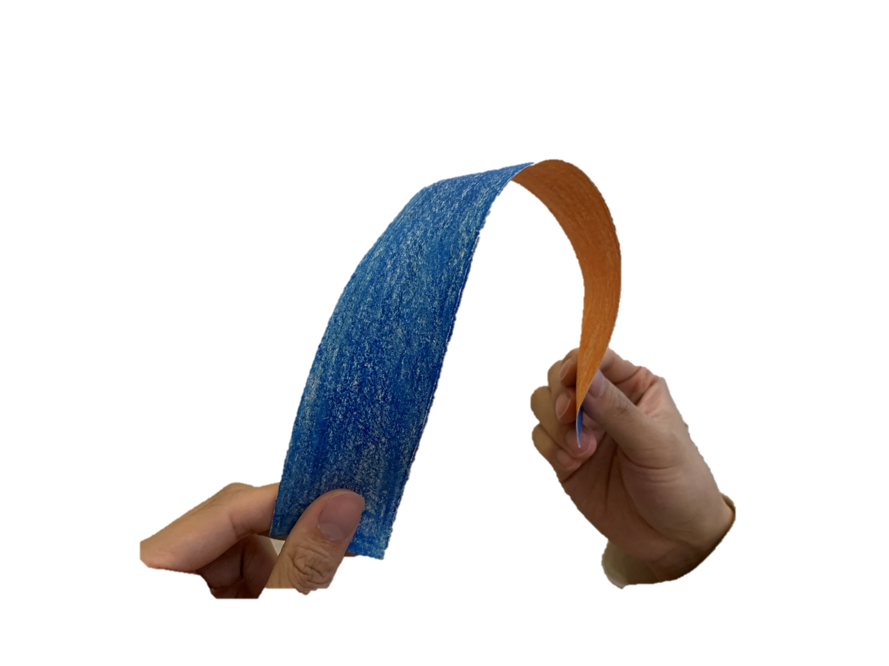Preprint Article Version 1 Preserved in Portico This version is not peer-reviewed

# Morphological Transformation of Arched Ribbon Driven by Torsion

Version 1 : Received: 18 April 2021 / Approved: 20 April 2021 / Online: 20 April 2021 (11:44:07 CEST)

How to cite: Dai, Y.; sun, B.; Zhang, Y.; Li, X. Morphological Transformation of Arched Ribbon Driven by Torsion. Preprints 2021, 2021040536 (doi: 10.20944/preprints202104.0536.v1). Dai, Y.; sun, B.; Zhang, Y.; Li, X. Morphological Transformation of Arched Ribbon Driven by Torsion. Preprints 2021, 2021040536 (doi: 10.20944/preprints202104.0536.v1).

## Abstract

The morphological transformation of an arched ribbon driven by torsion is a scientific problem that is connected with daily life and requires thorough analysis. An arched ribbon can achieve an instantaneous high speed through energy transformation and then return to the original shape of the structure. In this paper, based on the characteristics of the ribbon structure, the dynamic mathematical model of the arched ribbon driven by torsion is established from the Kirchhoff rod equation. The variations of the Euler angle of each point on the center line of the ribbon with the arc coordinate s and the rotation angle of the supports $\phi$ was examined. The relationship between the internal force distribution of each point in the direction of $\hat{d}_{a}$ and the material, cross-sectional properties, and rotation angle of the supports was obtained. We used ABAQUS, a nonlinear finite element analysis tool, to simulate the morphological transformations of the ribbons, verified our theory with simulation results, and reproduced the experimental results of Sano. Furthermore, we redefined the concept of the critical flipping point'' of Sano. In this paper, the dimensional analysis method was used to fit the simulation data. The following relationship between the critical width $w ^{*}$, thickness h, and the radius R of the ribbon with different cross sections was obtained: $w^{*}=A\cdot R\left(h/R\right)^{0.6}$, where A is 3.19 for rectangular cross sections and 3.06 for elliptical cross sections. By analyzing the simulation data, we determined the variation behavior of the out-of-plane deflection of the center point of the ribbon with the radius R, width w, and thickness h. Our research has guiding significance for understanding and designing arched ribbons driven by torsion, and the results can be applied to problems of different scales.## Keywords

ribbon, morphological transformation, torsion , critical width

## Subject

PHYSICAL SCIENCES, Acoustics

Views 0